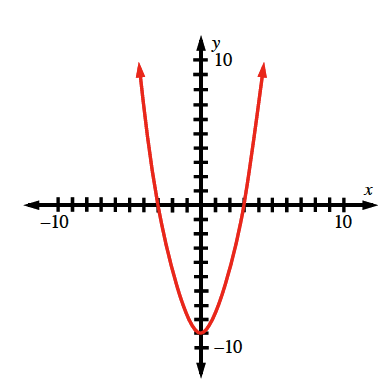### Home > AC > Chapter 8 > Lesson 8.1.2 > Problem8-18

8-18.

Graph $y = x^2 − 9$ on graph paper.

1. Name the $y$-intercept. What is the connection between the $y$-intercept and the rule $y = x^2 − 9$?

2. Name the $x$-intercepts. What is the connection between the $x$-intercepts and the rule $y = x^2 − 9$?

The $y$-intercept is where the parabola intersects the
$y$-axis.

The $y$-intercept is where $x=0$.
$y = (0)^² − 9$
$y=−9$

The $x$-intercept is where the parabola intersects the
$x$-axis.

The $x$-intercept is where $y=0$
$0 = x^² − 9$
$9 = x^²$

$x=−3,+3$

${\sqrt{9} = x}$